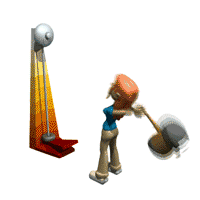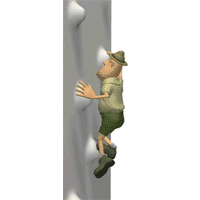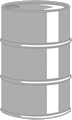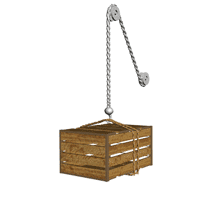Try this at home!

Water that won't boil!

Turning which gear would give youWilliam Dwight Whitney The Century Dictionary and Cyclopedia: An encyclopedic Lexicon of the English Language. (New York: The Century Co., 1889)4499

Small Gear

C) Power - rate of doing work or expending energyP = W/t = Fd/t = Fv (watt) = (J/s) (N)m/s

Scalar

1 Horse Power = 746 WattsEx 1) 1440 kg car accelerates from rest to 95 km/hr in 7.4 sec. What average power is used?P = W/t

P = DKE/t

= 1/2m(V22 - V12)/t

Convert km/hr

95 km/hr[1000m/km][hr/3600sec]

6.6 x 104 W or 88 hp

1 Horse Power = 746 WattsP = W/t = Fd/t = Fv (watt) = (J/s) (N)m/sEx 2) A 95 kg football player runs up stairs in 66s. The stairs are 140 m long at an angle of 32°   Her power output vertically isP = W/t

P = Fd/t

F = [mgsinq]d/t

P = 1047 W

P = 1.0 x 103 W

 P = W/t = Fd/t = Fv (watt) = (J/s) (N)m/s

P = Fd/tEx 3)  1200 kg car has a max. power output of 120 hp. How steep a hill can it climb at constant speed of 75 km/hr if the frictional force is 650 N?

v=75 km/hr[1000m/km][hr/3600s]

v = 21 m/s

P = Fv

1 Horse Power = 746 Watts

P = 746 W[120 hp]

P = 9.0 x 105 W

At constant speed a = 0

Fnet = -mgsinq - f + Fcar = 0

Fcar = mgsinq + f

P = Fv

P = [mgsinq + f]v

= 18°

 P = W/t = Fd/t = Fv (watt) = (J/s) (N)m/s